# RD Sharma Solutions For Class 7 Maths Exercise 3.3 Chapter 3 Decimals

The pdf of RD Sharma Solutions for Exercise 3.3 of Class 7 Maths Chapter 3 Decimals are provided here. The questions present have been solved by BYJU’S experts in Maths, and this will help students solve the problems without any difficulties. This exercise contains ten questions with many sub-questions. By practising sincerely, the students can obtain worthy results in Class 7 exams by using the RD Sharma Solutions. By practising the RD Sharma Solutions for Class 7, students will be thorough about their concepts. This exercise deals with the division of decimals.

## Download the PDF of RD Sharma Solutions For Class 7 Maths Chapter 3 – Decimals Exercise 3.3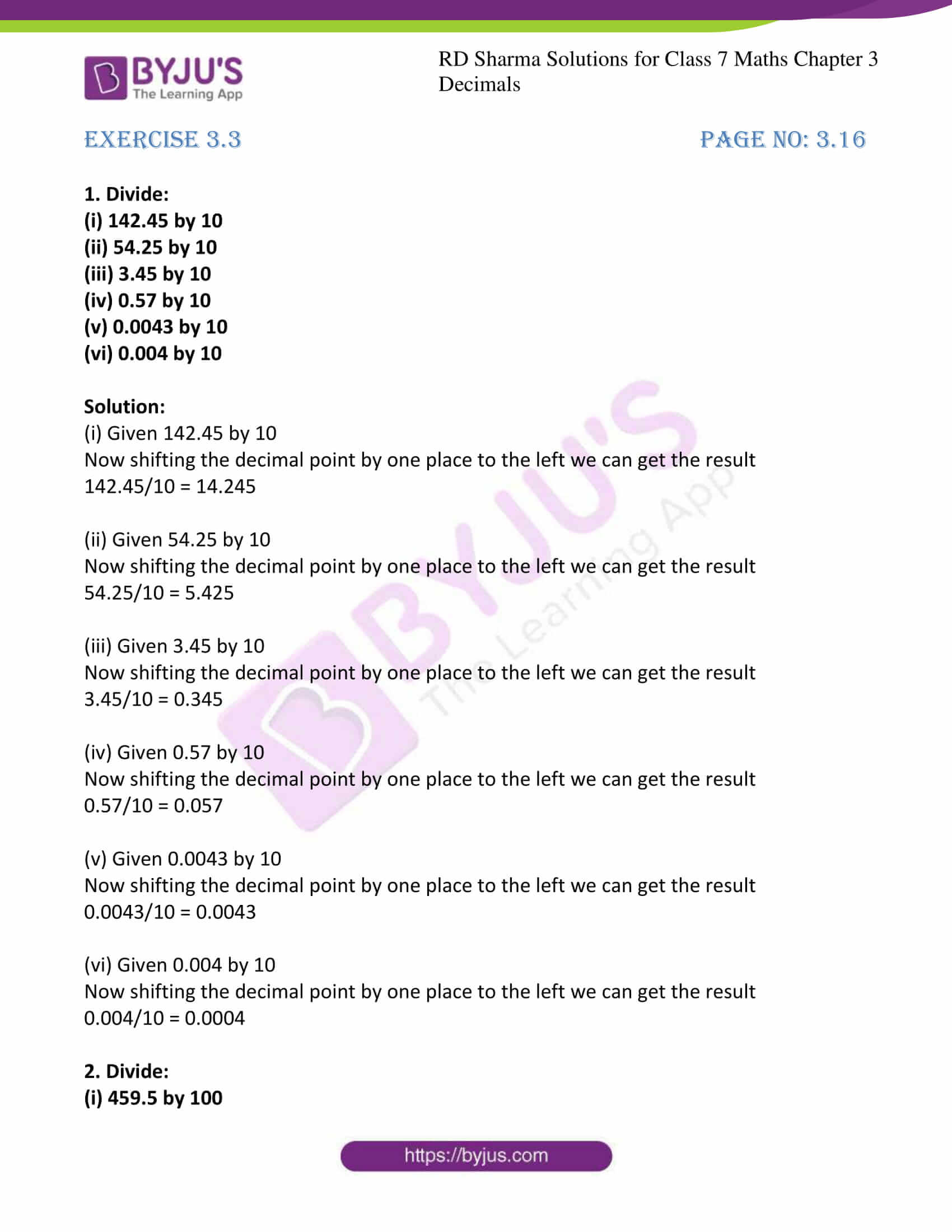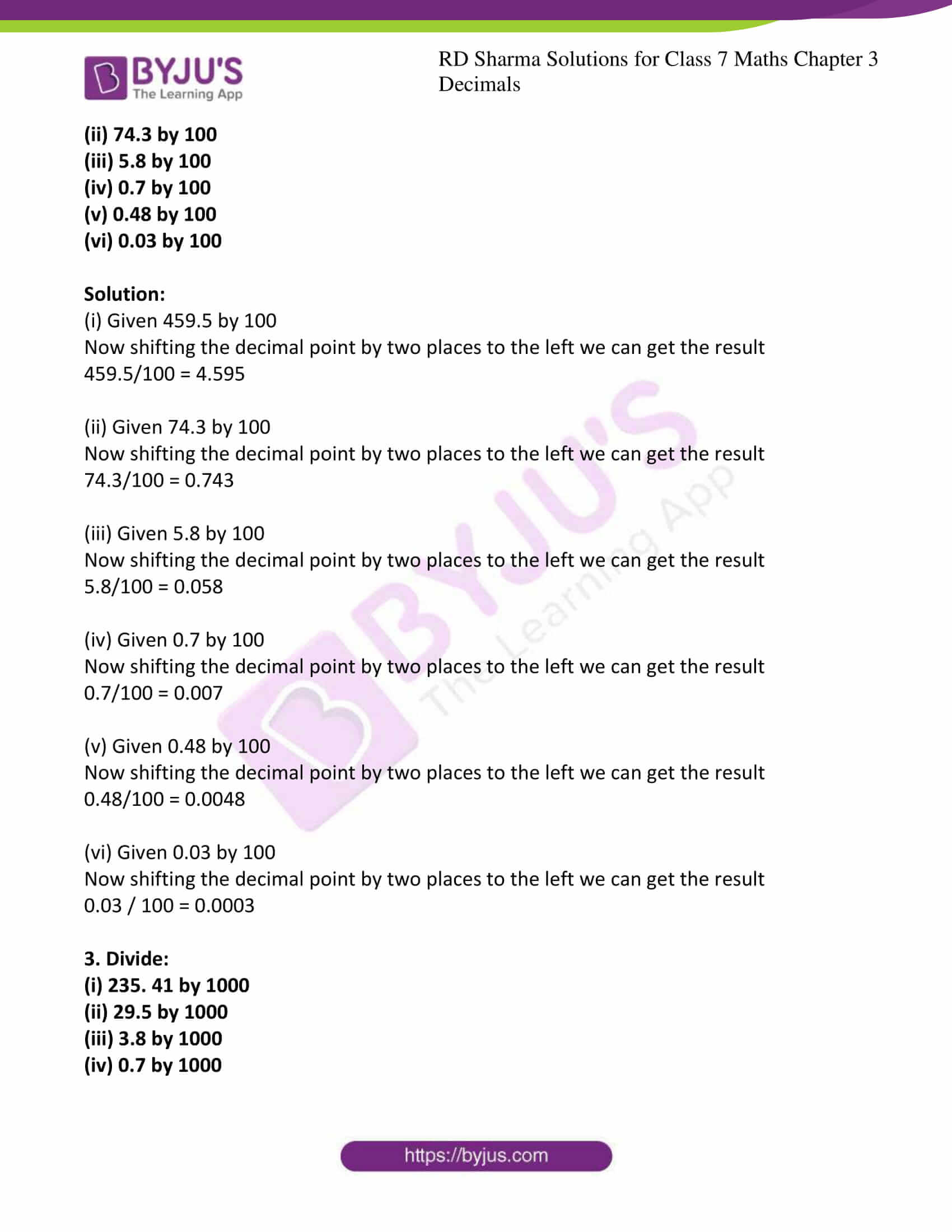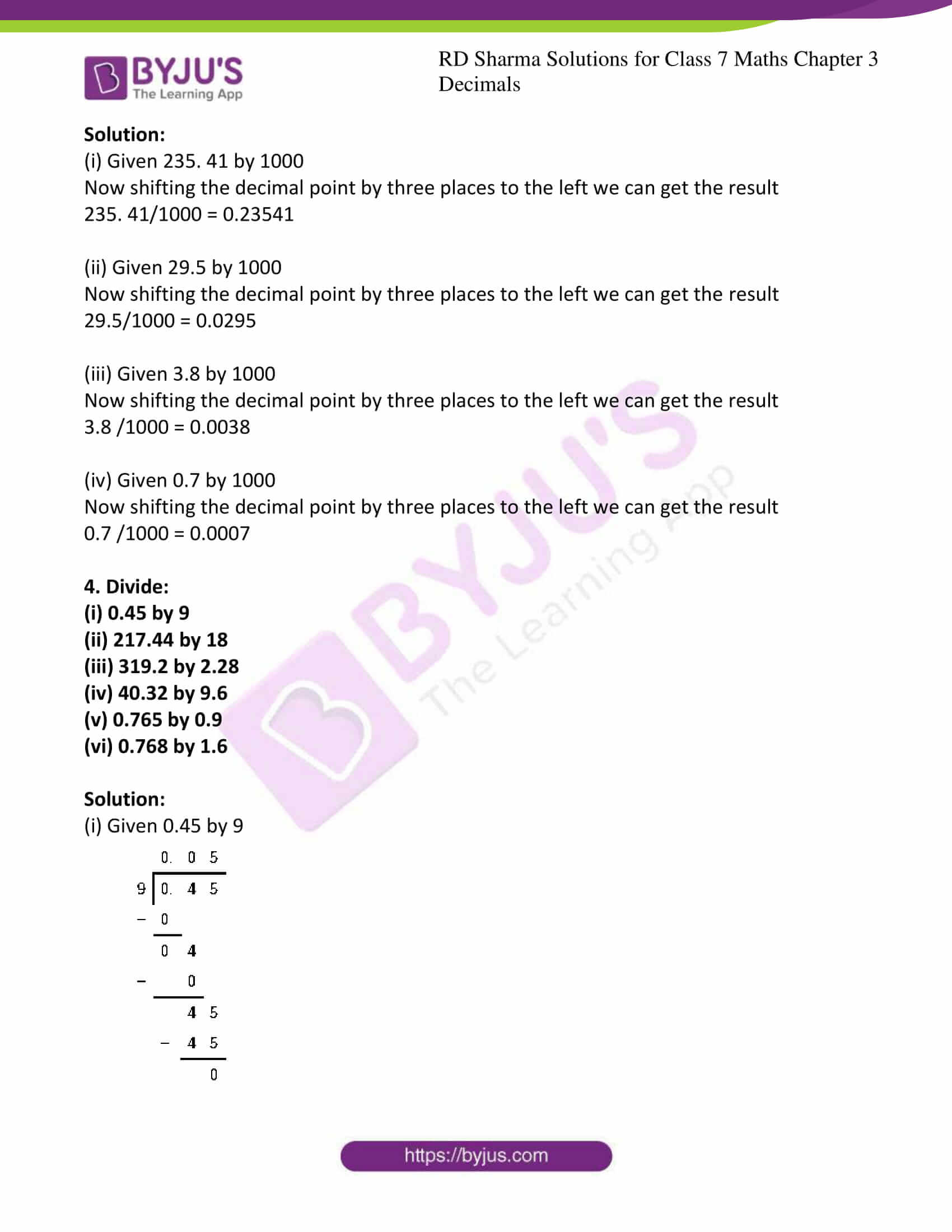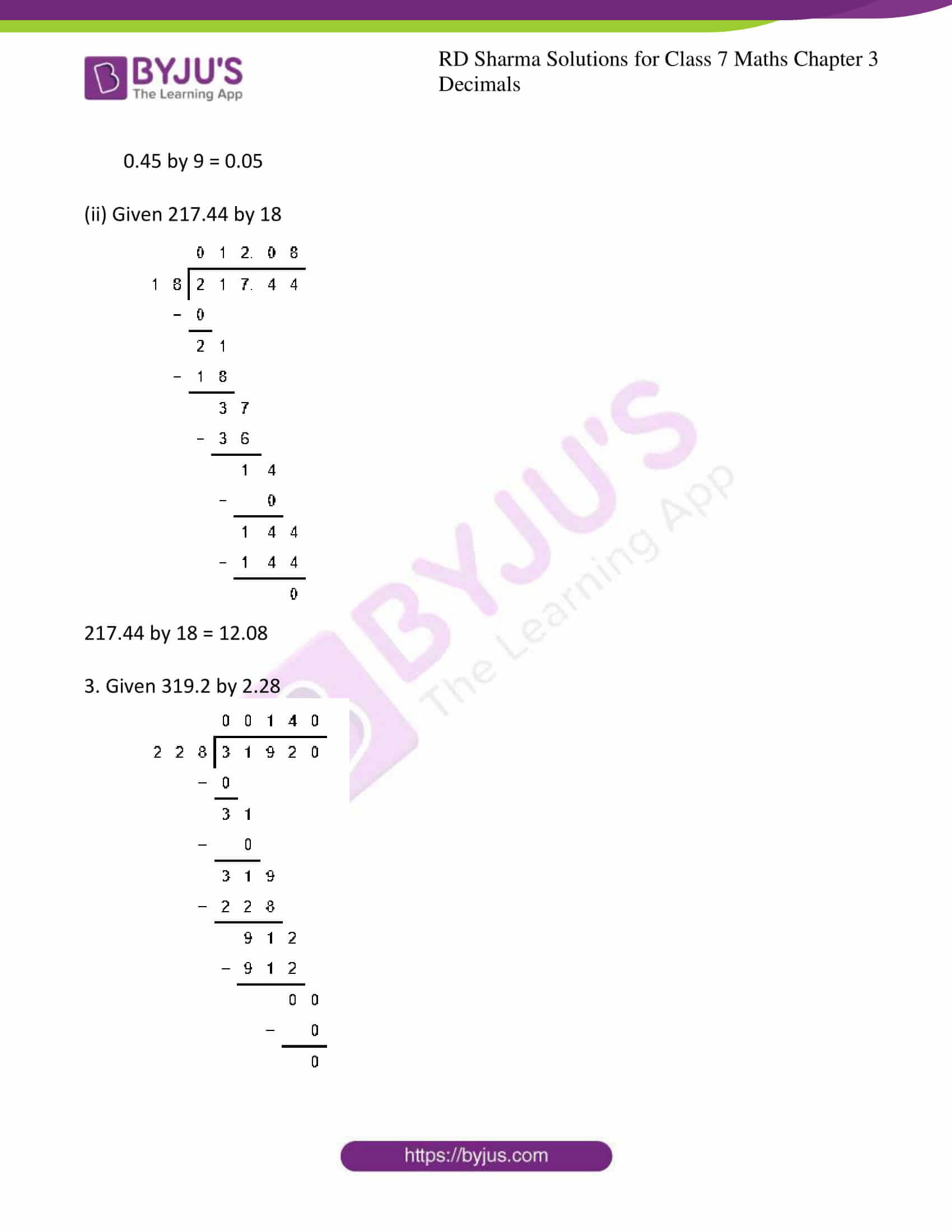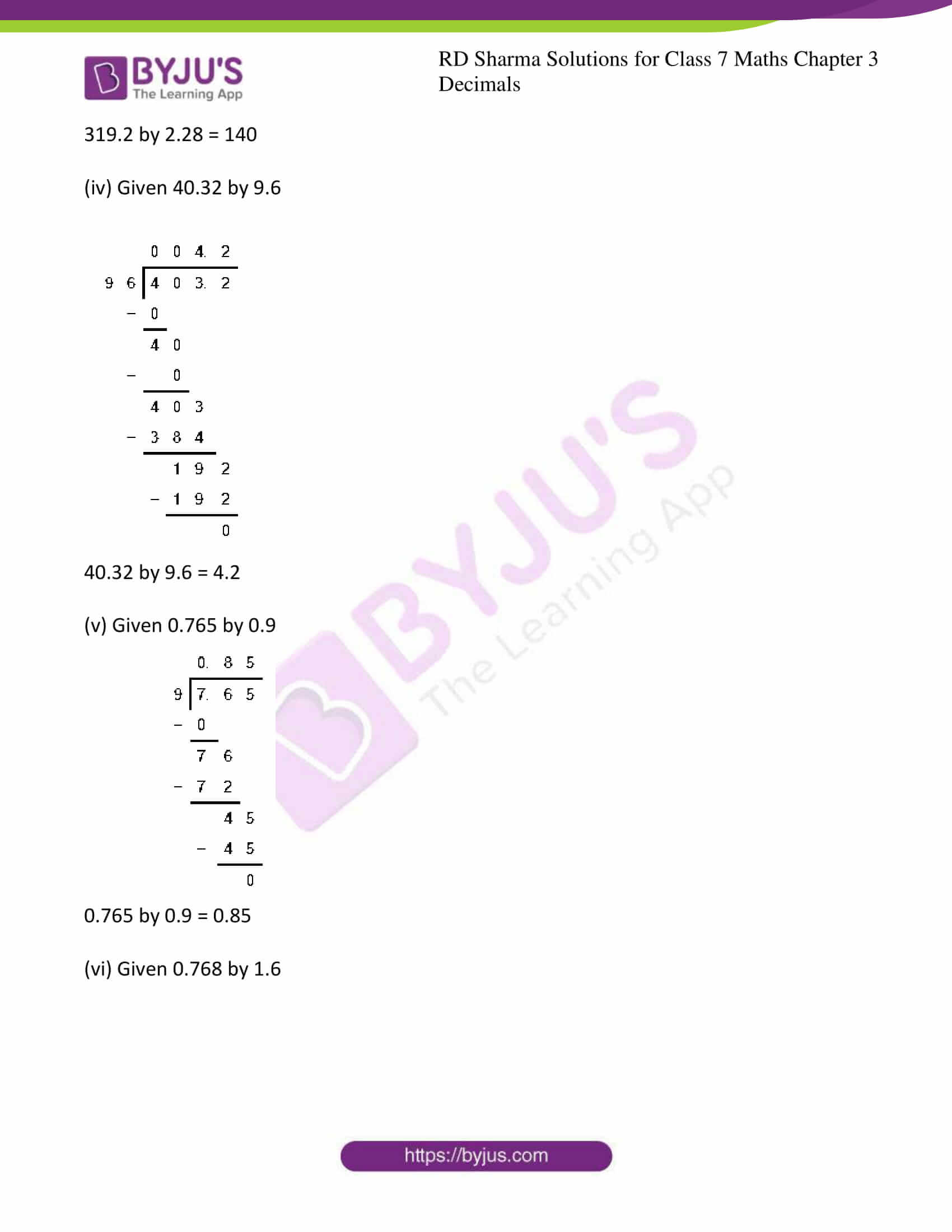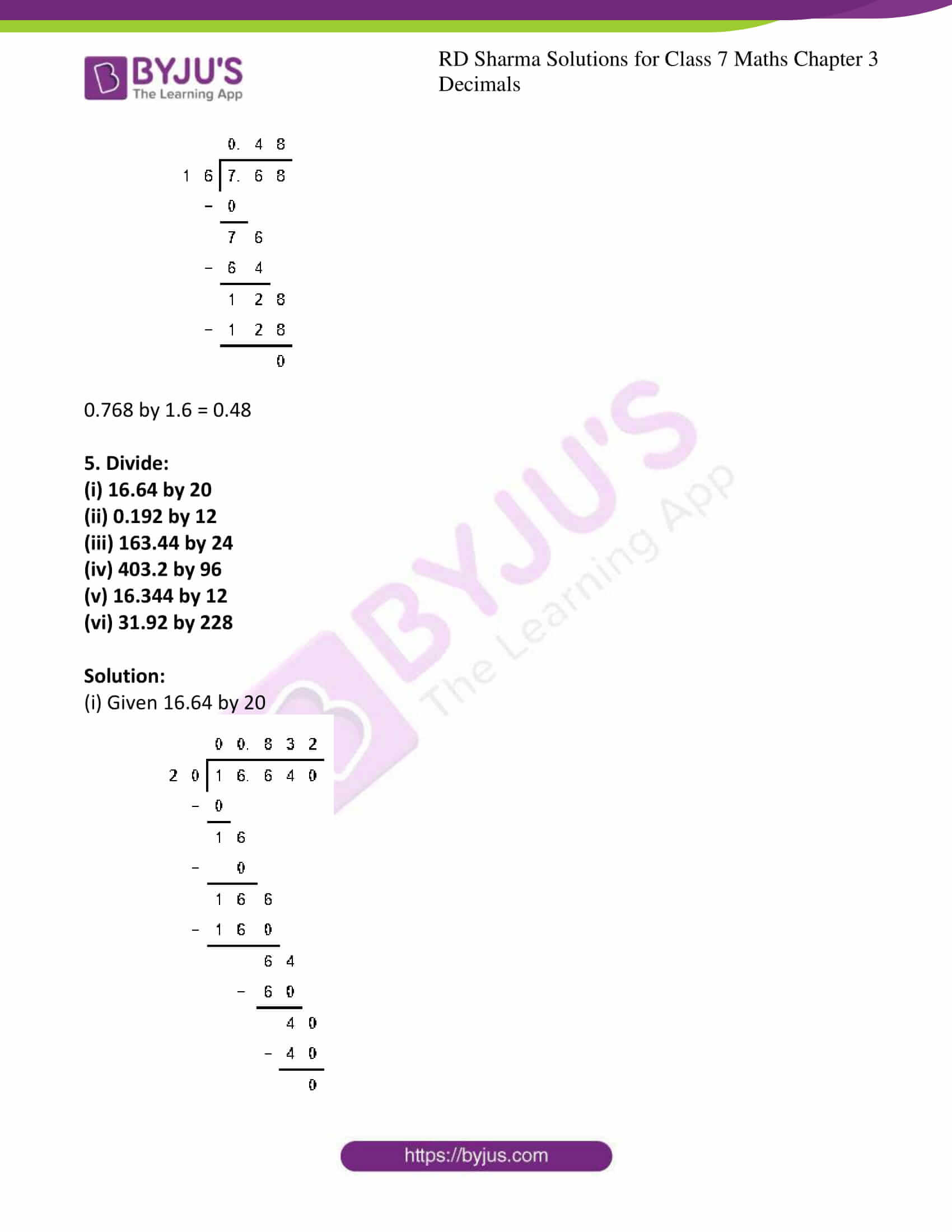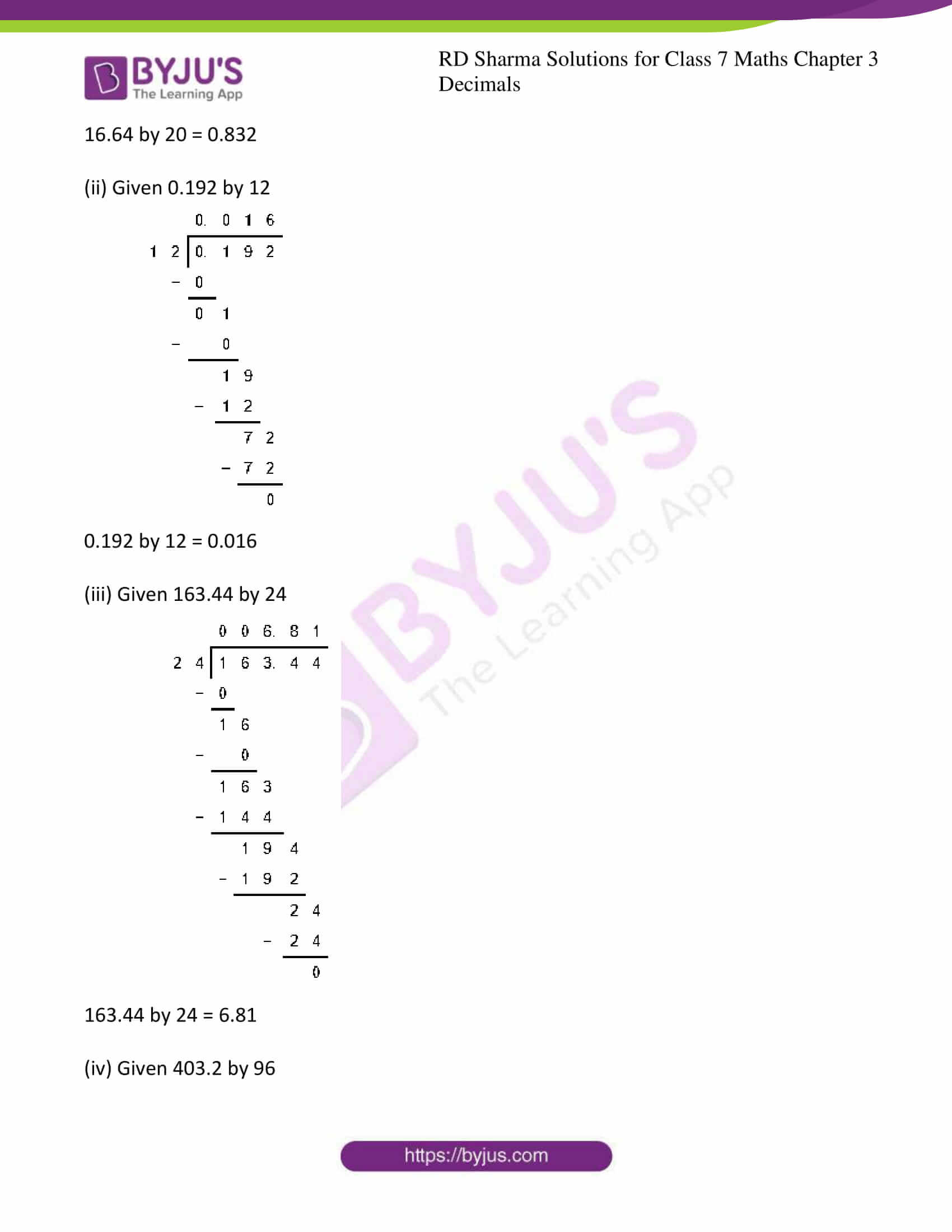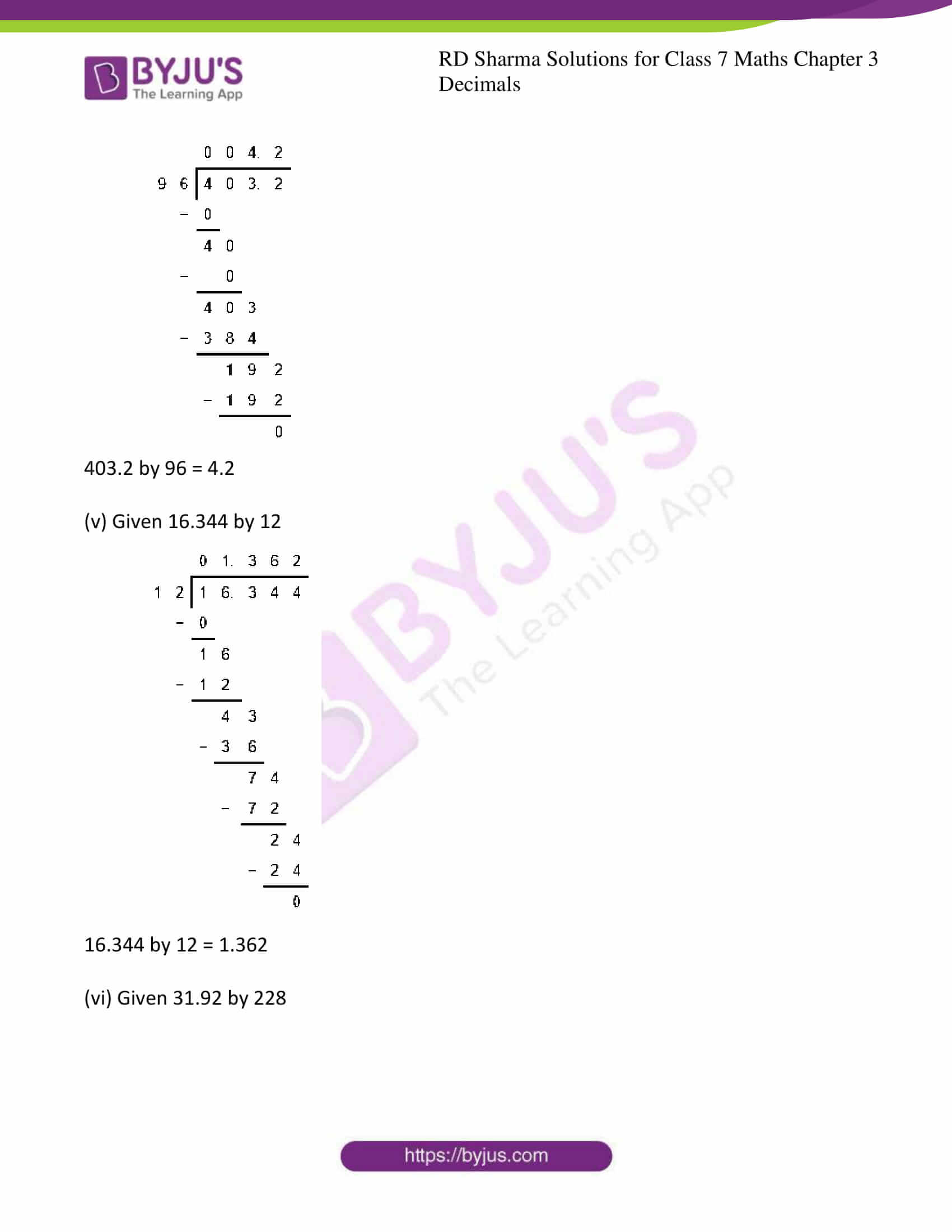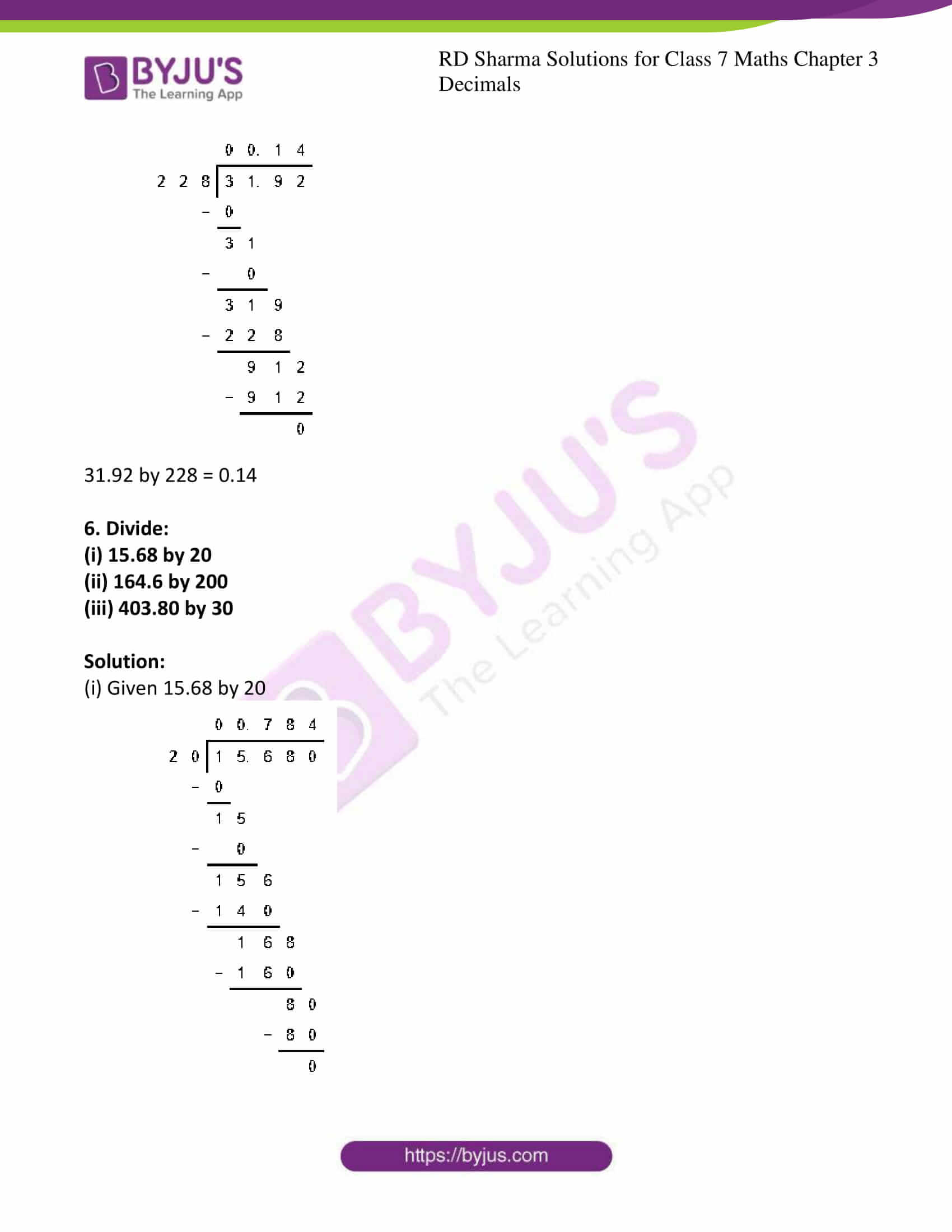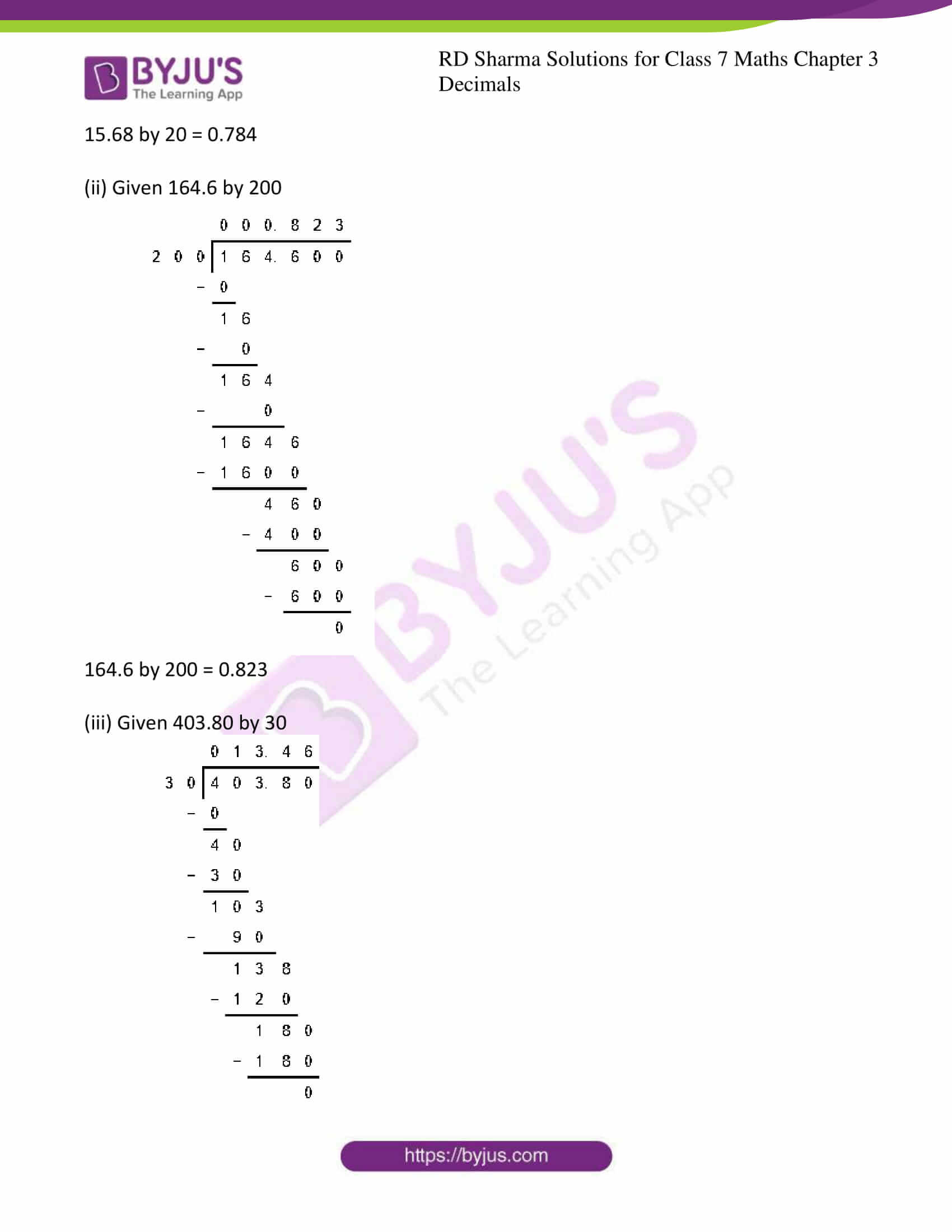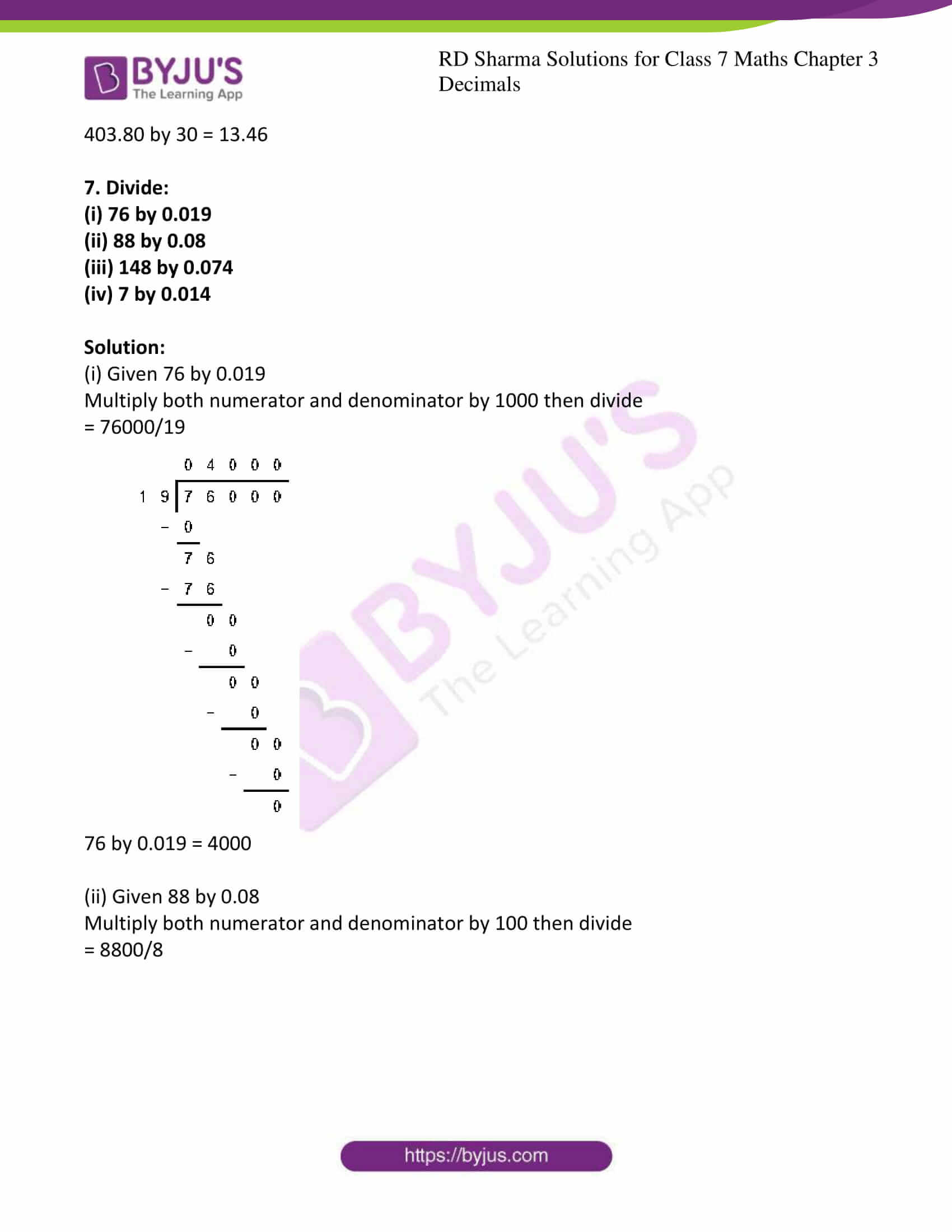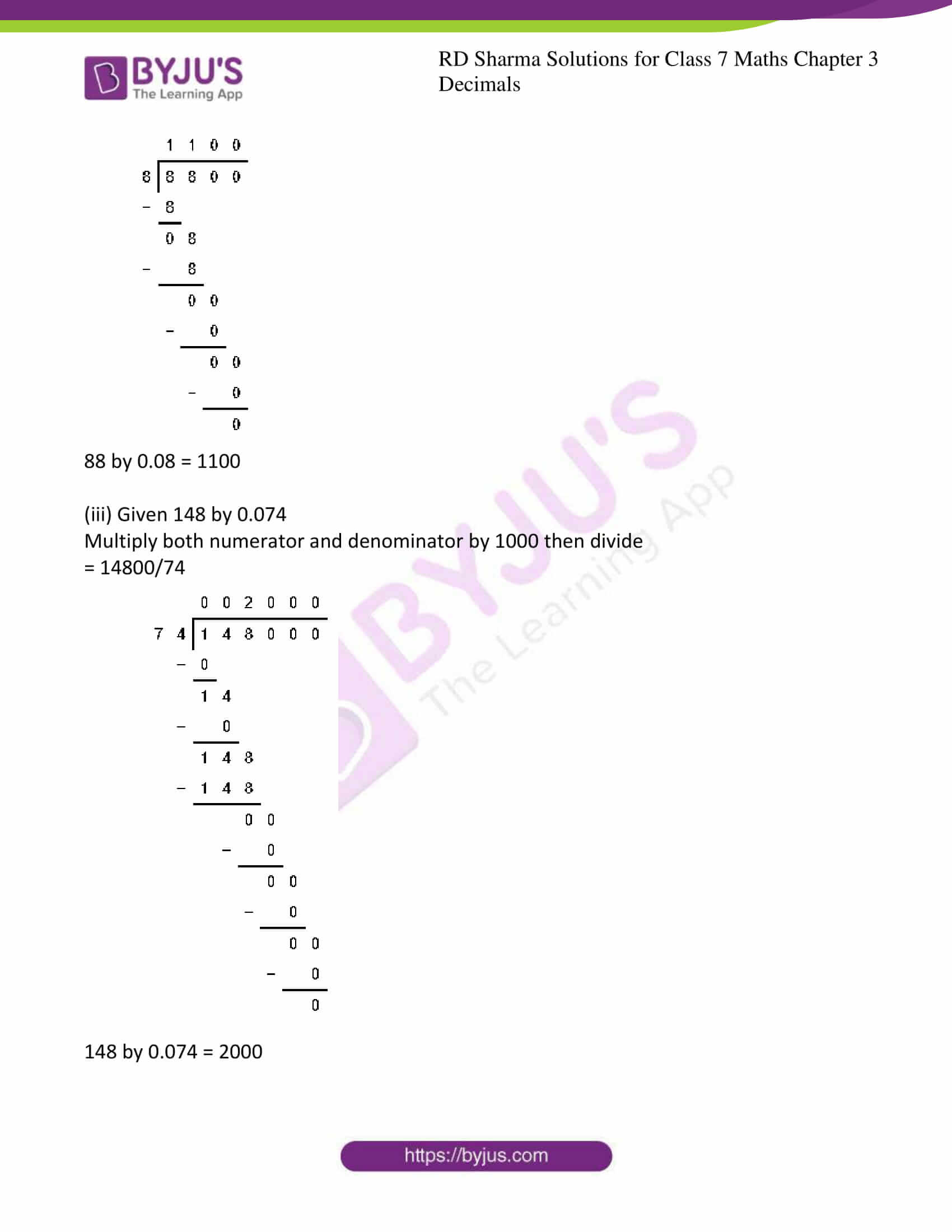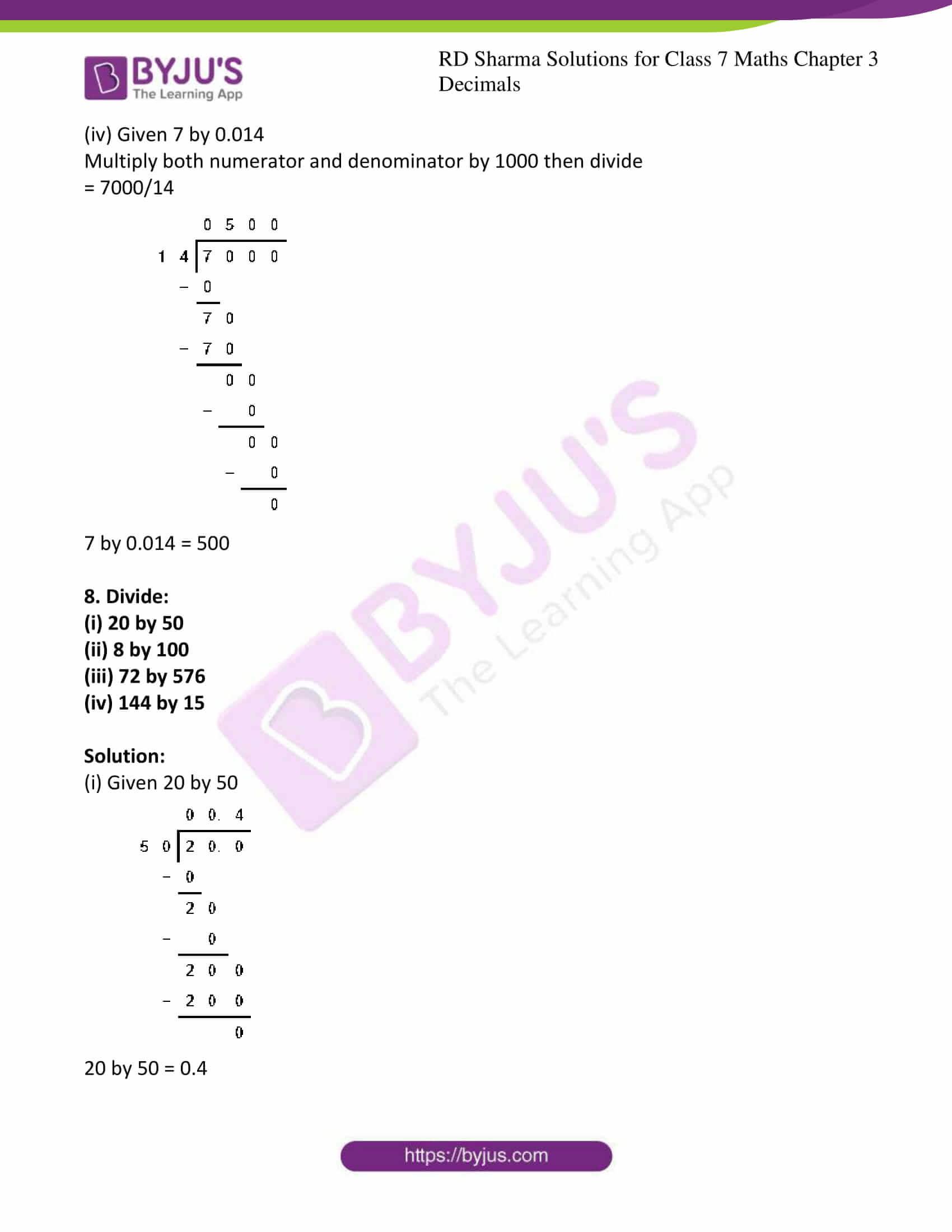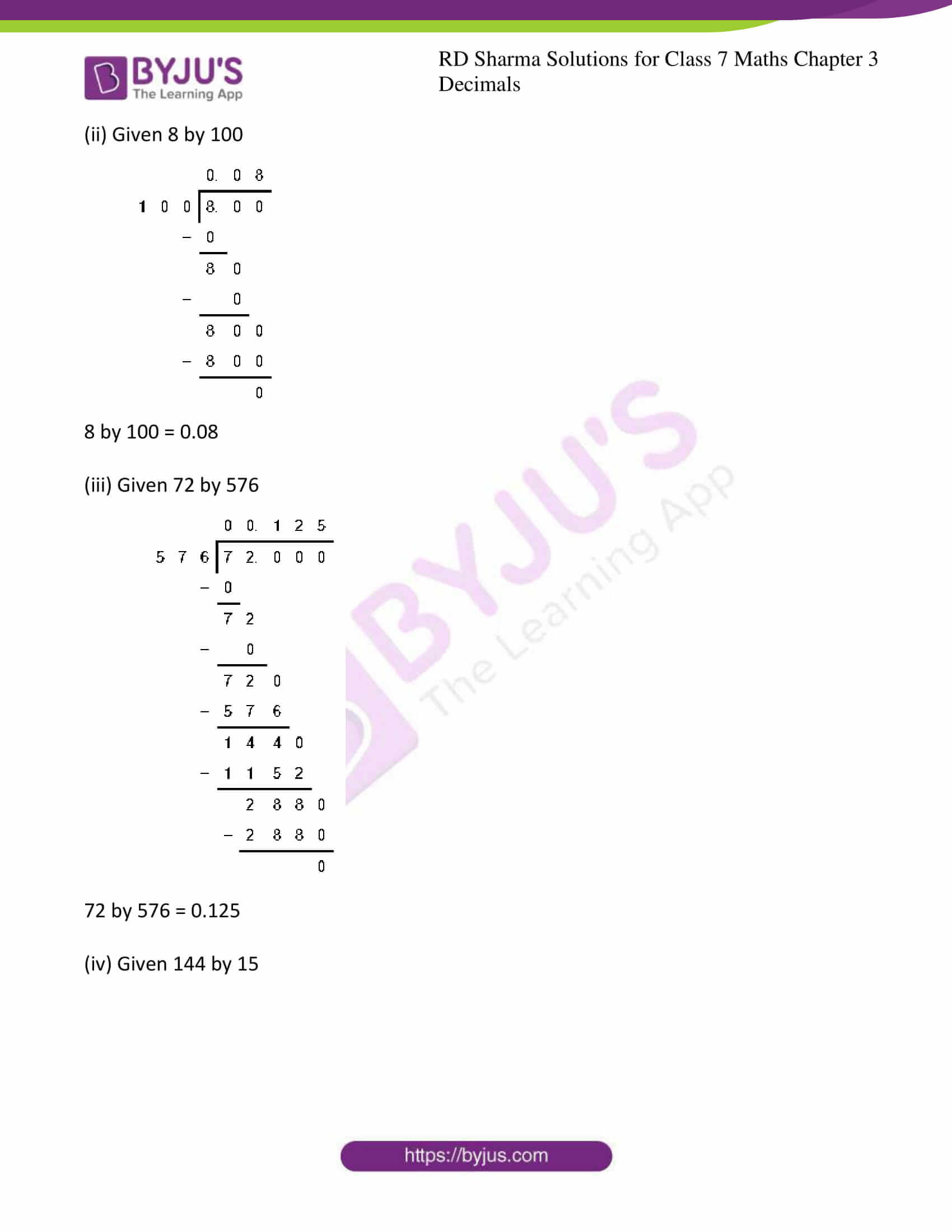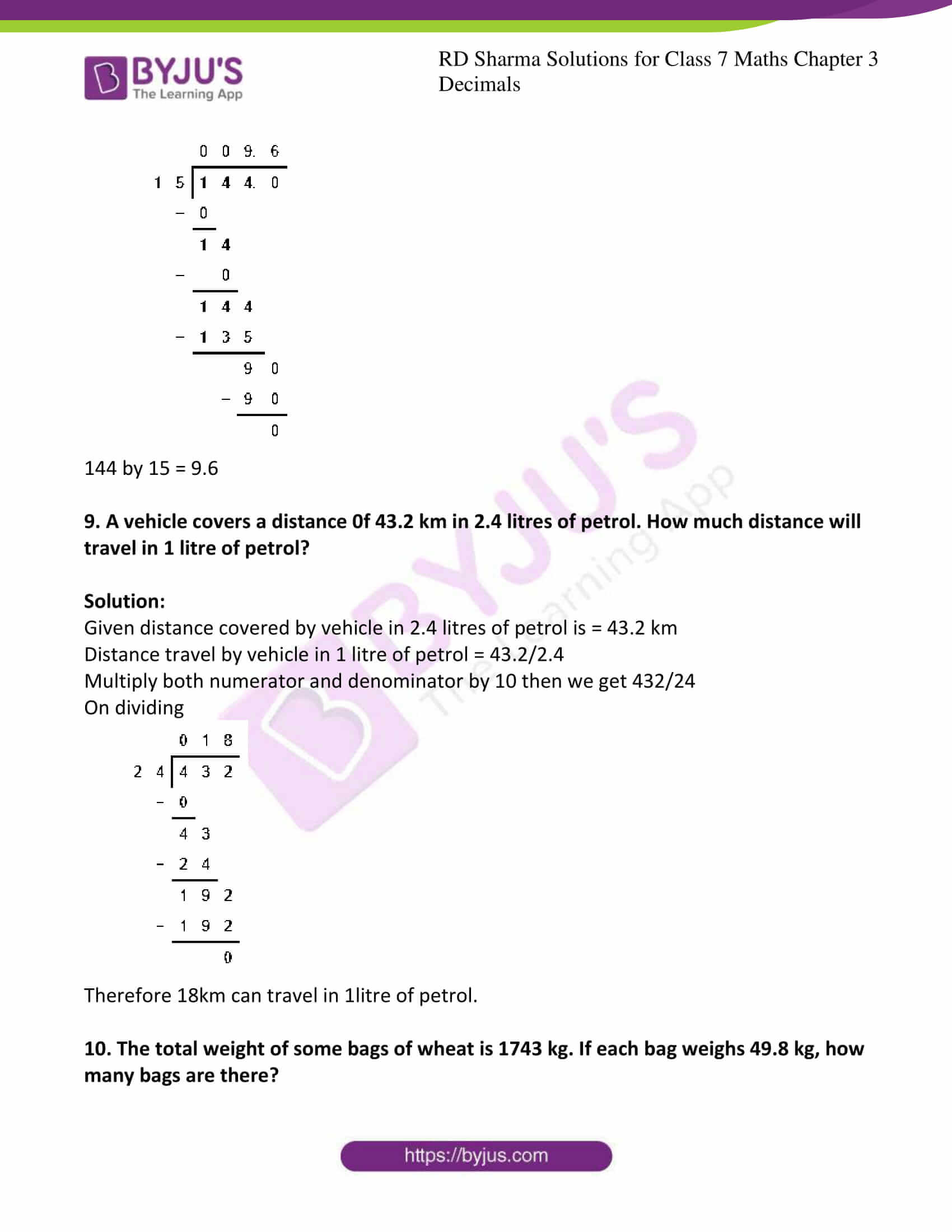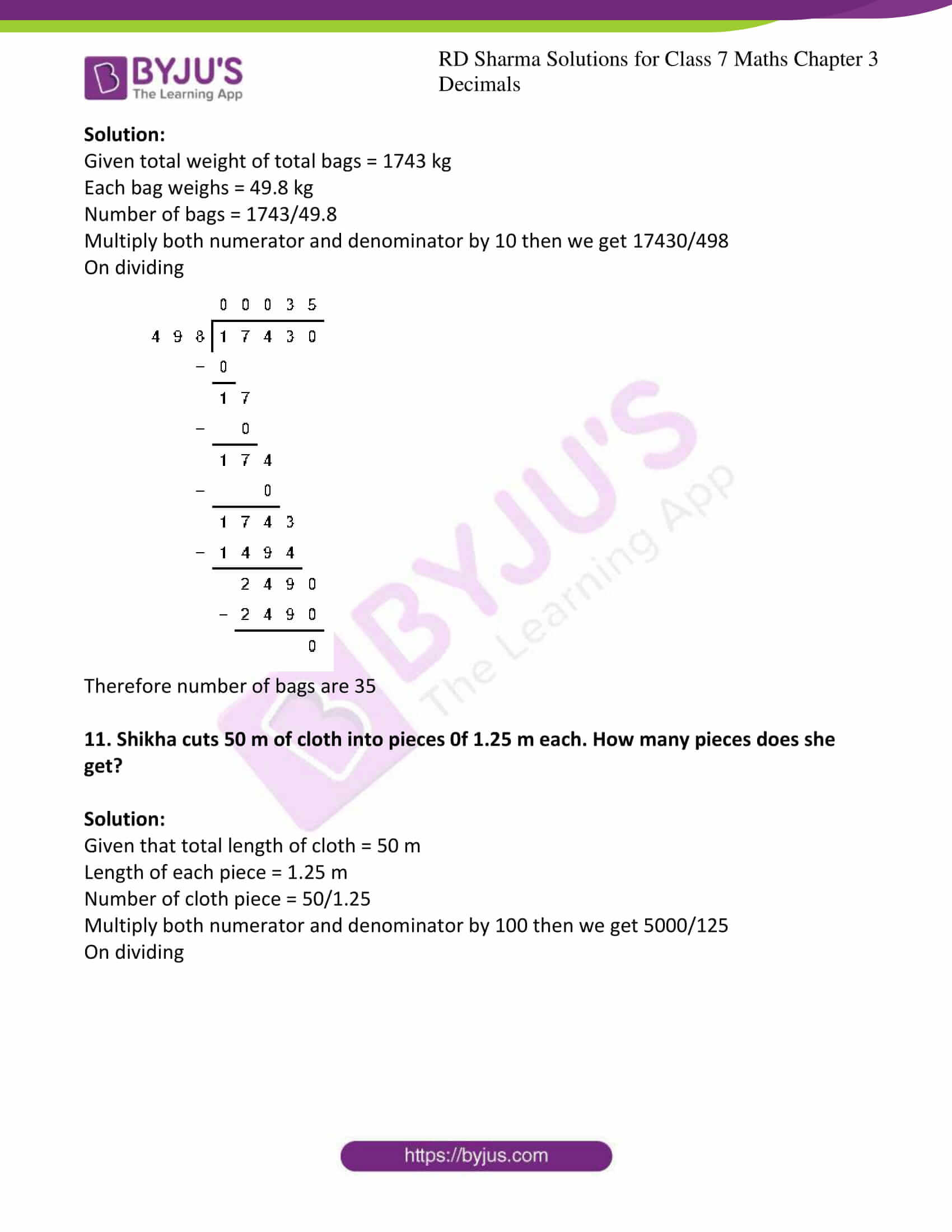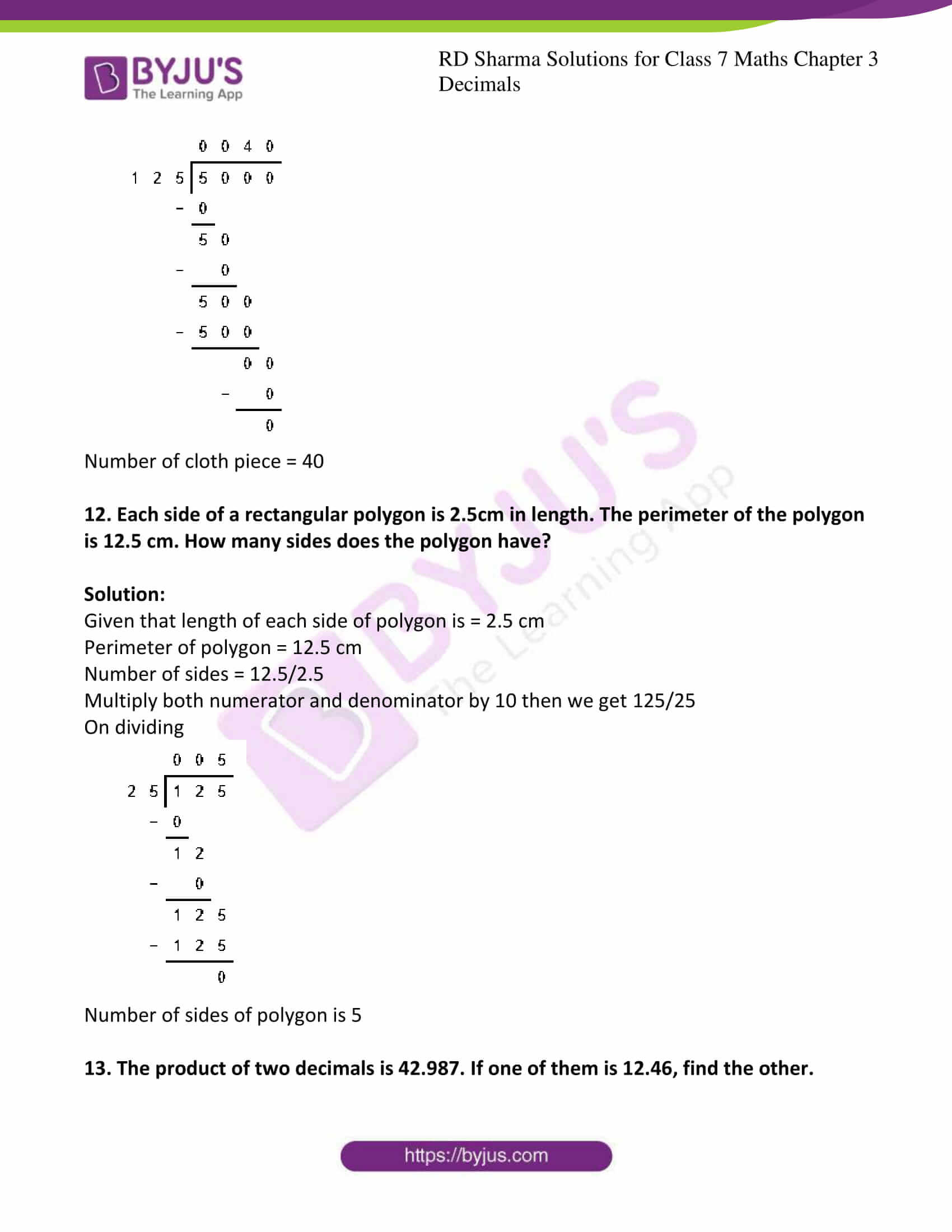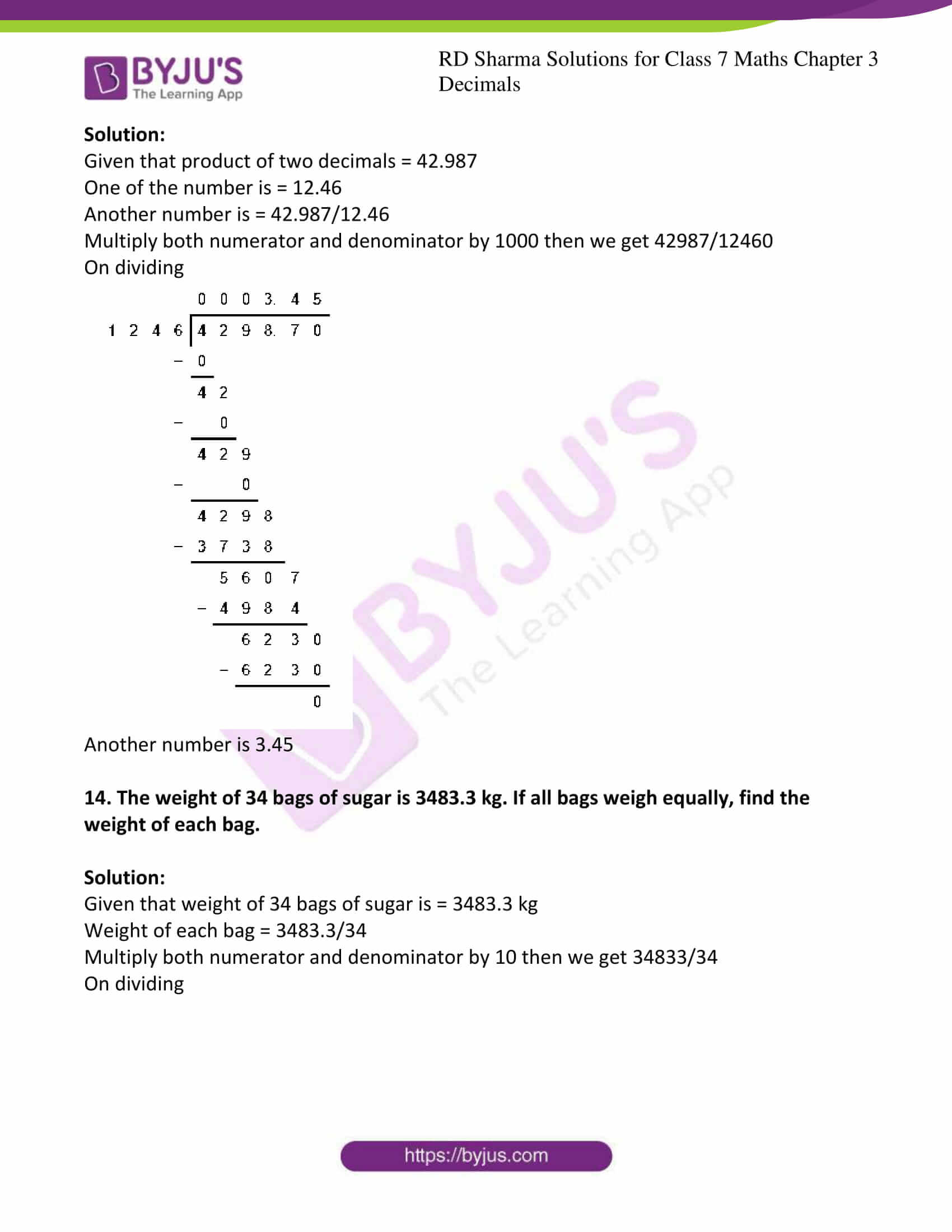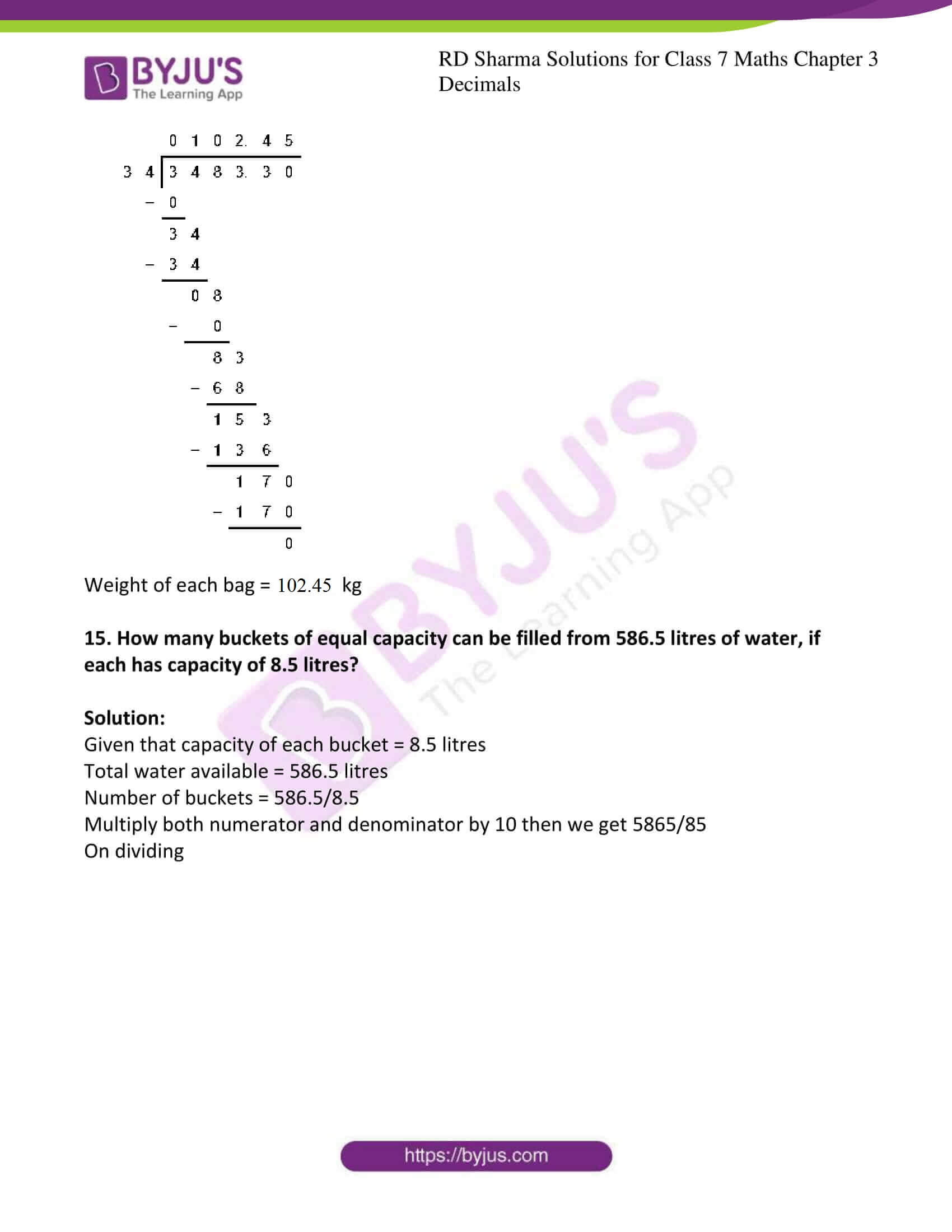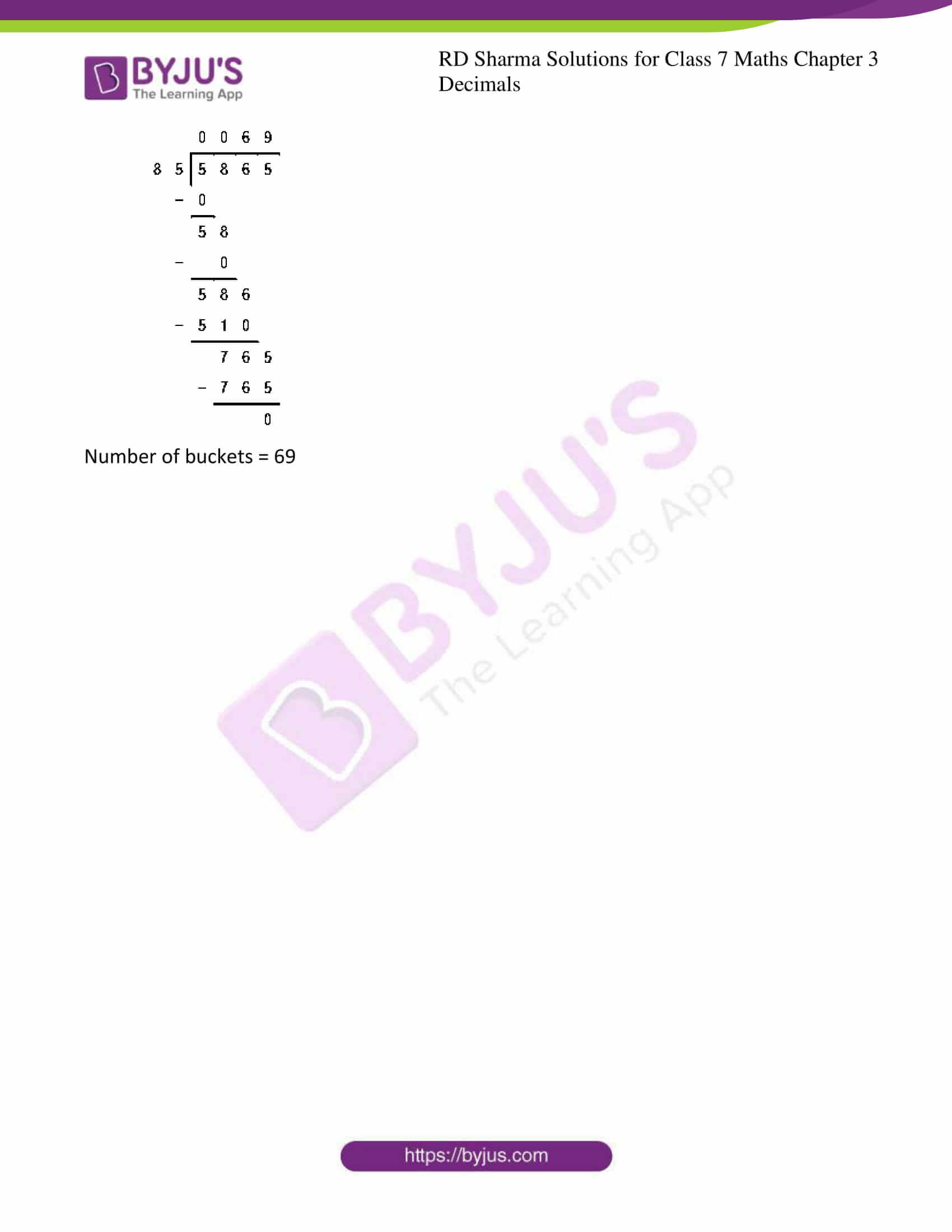### Access answers to Maths RD Sharma Solutions For Class 7 Chapter 3 – Decimals Exercise 3.3

1. Divide:

(i) 142.45 by 10

(ii) 54.25 by 10

(iii) 3.45 by 10

(iv) 0.57 by 10

(v) 0.0043 by 10

(vi) 0.004 by 10

Solution:

(i) Given 142.45 by 10

Now shifting the decimal point by one place to the left we can get the result

142.45/10 = 14.245

(ii) Given 54.25 by 10

Now shifting the decimal point by one place to the left we can get the result

54.25/10 = 5.425

(iii) Given 3.45 by 10

Now shifting the decimal point by one place to the left we can get the result

3.45/10 = 0.345

(iv) Given 0.57 by 10

Now shifting the decimal point by one place to the left we can get the result

0.57/10 = 0.057

(v) Given 0.0043 by 10

Now shifting the decimal point by one place to the left we can get the result

0.0043/10 = 0.0043

(vi) Given 0.004 by 10

Now shifting the decimal point by one place to the left we can get the result

0.004/10 = 0.0004

2. Divide:

(i) 459.5 by 100

(ii) 74.3 by 100

(iii) 5.8 by 100

(iv) 0.7 by 100

(v) 0.48 by 100

(vi) 0.03 by 100

Solution:

(i) Given 459.5 by 100

Now shifting the decimal point by two places to the left we can get the result

459.5/100 = 4.595

(ii) Given 74.3 by 100

Now shifting the decimal point by two places to the left we can get the result

74.3/100 = 0.743

(iii) Given 5.8 by 100

Now shifting the decimal point by two places to the left we can get the result

5.8/100 = 0.058

(iv) Given 0.7 by 100

Now shifting the decimal point by two places to the left we can get the result

0.7/100 = 0.007

(v) Given 0.48 by 100

Now shifting the decimal point by two places to the left we can get the result

0.48/100 = 0.0048

(vi) Given 0.03 by 100

Now shifting the decimal point by two places to the left we can get the result

0.03 / 100 = 0.0003

3. Divide:

(i) 235. 41 by 1000

(ii) 29.5 by 1000

(iii) 3.8 by 1000

(iv) 0.7 by 1000

Solution:

(i) Given 235. 41 by 1000

Now shifting the decimal point by three places to the left we can get the result

235. 41/1000 = 0.23541

(ii) Given 29.5 by 1000

Now shifting the decimal point by three places to the left we can get the result

29.5/1000 = 0.0295

(iii) Given 3.8 by 1000

Now shifting the decimal point by three places to the left we can get the result

3.8 /1000 = 0.0038

(iv) Given 0.7 by 1000

Now shifting the decimal point by three places to the left we can get the result

0.7 /1000 = 0.0007

4. Divide:

(i) 0.45 by 9

(ii) 217.44 by 18

(iii) 319.2 by 2.28

(iv) 40.32 by 9.6

(v) 0.765 by 0.9

(vi) 0.768 by 1.6

Solution:

(i) Given 0.45 by 9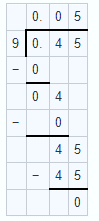0.45 by 9 = 0.05

(ii) Given 217.44 by 18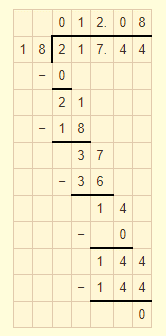217.44 by 18 = 12.08

3. Given 319.2 by 2.28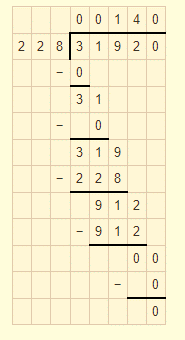319.2 by 2.28 = 140

(iv) Given 40.32 by 9.6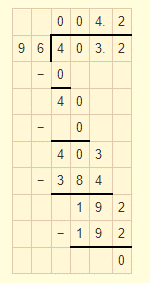40.32 by 9.6 = 4.2

(v) Given 0.765 by 0.9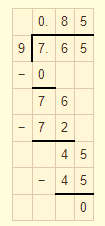0.765 by 0.9 = 0.85

(vi) Given 0.768 by 1.6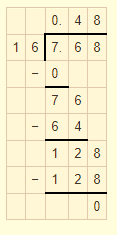0.768 by 1.6 = 0.48

5. Divide:

(i) 16.64 by 20

(ii) 0.192 by 12

(iii) 163.44 by 24

(iv) 403.2 by 96

(v) 16.344 by 12

(vi) 31.92 by 228

Solution:

(i) Given 16.64 by 20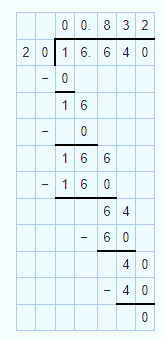16.64 by 20 = 0.832

(ii) Given 0.192 by 12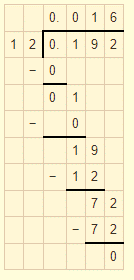0.192 by 12 = 0.016

(iii) Given 163.44 by 24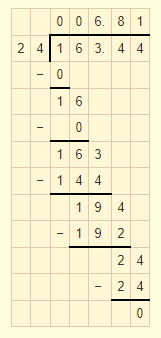163.44 by 24 = 6.81

(iv) Given 403.2 by 96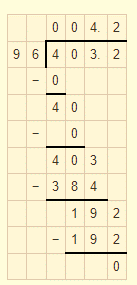403.2 by 96 = 4.2

(v) Given 16.344 by 12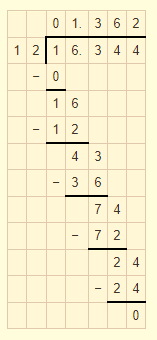16.344 by 12 = 1.362

(vi) Given 31.92 by 228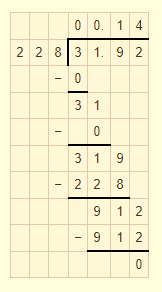31.92 by 228 = 0.14

6. Divide:

(i) 15.68 by 20

(ii) 164.6 by 200

(iii) 403.80 by 30

Solution:

(i) Given 15.68 by 20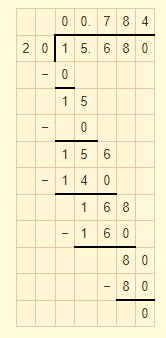15.68 by 20 = 0.784

(ii) Given 164.6 by 200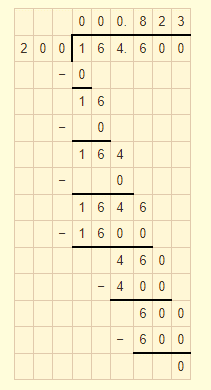164.6 by 200 = 0.823

(iii) Given 403.80 by 30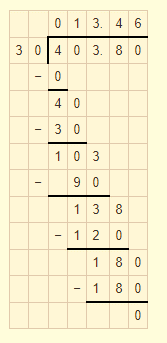403.80 by 30 = 13.46

7. Divide:

(i) 76 by 0.019

(ii) 88 by 0.08

(iii) 148 by 0.074

(iv) 7 by 0.014

Solution:

(i) Given 76 by 0.019

Multiply both numerator and denominator by 1000 then divide

= 76000/19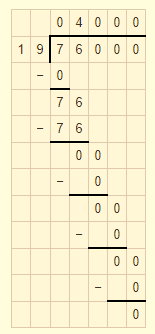76 by 0.019 = 4000

(ii) Given 88 by 0.08

Multiply both numerator and denominator by 100 then divide

= 8800/8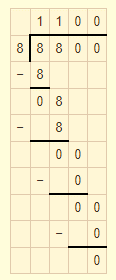88 by 0.08 = 1100

(iii) Given 148 by 0.074

Multiply both numerator and denominator by 1000 then divide

= 14800/74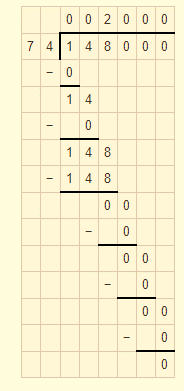148 by 0.074 = 2000

(iv) Given 7 by 0.014

Multiply both numerator and denominator by 1000 then divide

= 7000/14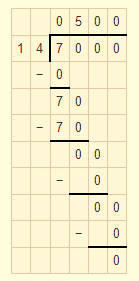7 by 0.014 = 500

8. Divide:

(i) 20 by 50

(ii) 8 by 100

(iii) 72 by 576

(iv) 144 by 15

Solution:

(i) Given 20 by 50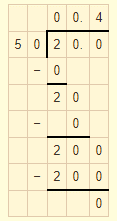20 by 50 = 0.4

(ii) Given 8 by 100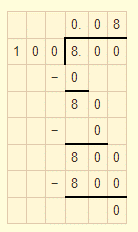8 by 100 = 0.08

(iii) Given 72 by 576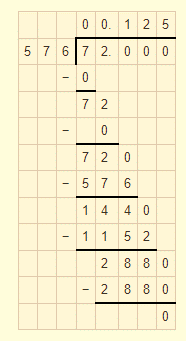72 by 576 = 0.125

(iv) Given 144 by 15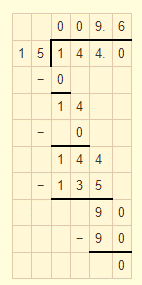144 by 15 = 9.6

9. A vehicle covers a distance 0f 43.2 km in 2.4 litres of petrol. How much distance will travel in 1 litre of petrol?

Solution:

Given distance covered by vehicle in 2.4 litres of petrol is = 43.2 km

Distance travel by vehicle in 1 litre of petrol = 43.2/2.4

Multiply both numerator and denominator by 10 then we get 432/24

On dividing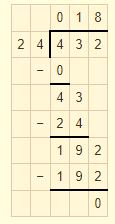Therefore 18km can travel in 1litre of petrol.

10. The total weight of some bags of wheat is 1743 kg. If each bag weighs 49.8 kg, how many bags are there?

Solution:

Given total weight of total bags = 1743 kg

Each bag weighs = 49.8 kg

Number of bags = 1743/49.8

Multiply both numerator and denominator by 10 then we get 17430/498

On dividing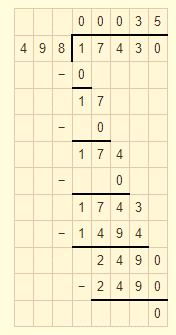Therefore number of bags are 35

11. Shikha cuts 50 m of cloth into pieces 0f 1.25 m each. How many pieces does she get?

Solution:

Given that total length of cloth = 50 m

Length of each piece = 1.25 m

Number of cloth piece = 50/1.25

Multiply both numerator and denominator by 100 then we get 5000/125

On dividing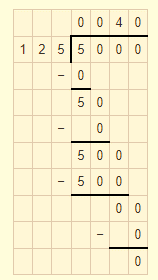Number of cloth piece = 40

12. Each side of a rectangular polygon is 2.5cm in length. The perimeter of the polygon is 12.5 cm. How many sides does the polygon have?

Solution:

Given that length of each side of polygon is = 2.5 cm

Perimeter of polygon = 12.5 cm

Number of sides = 12.5/2.5

Multiply both numerator and denominator by 10 then we get 125/25

On dividing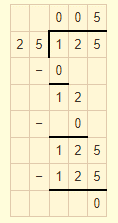Number of sides of polygon is 5

13. The product of two decimals is 42.987. If one of them is 12.46, find the other.

Solution:

Given that product of two decimals = 42.987

One of the number is = 12.46

Another number is = 42.987/12.46

Multiply both numerator and denominator by 1000 then we get 42987/12460

On dividing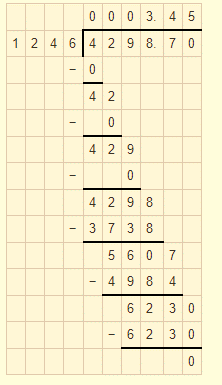Another number is 3.45

14. The weight of 34 bags of sugar is 3483.3 kg. If all bags weigh equally, find the weight of each bag.

Solution:

Given that weight of 34 bags of sugar is = 3483.3 kg

Weight of each bag = 3483.3/34

Multiply both numerator and denominator by 10 then we get 34833/34

On dividing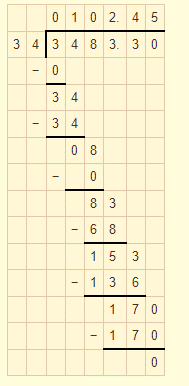Weight of each bag = 102.45 kg

15. How many buckets of equal capacity can be filled from 586.5 litres of water, if each has capacity of 8.5 litres?

Solution:

Given that capacity of each bucket = 8.5 litres

Total water available = 586.5 litres

Number of buckets = 586.5/8.5

Multiply both numerator and denominator by 10 then we get 5865/85

On dividing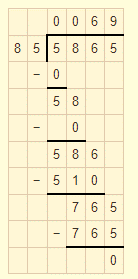Number of buckets = 69

### RD Sharma Solutions for Class 7 Maths Chapter – 3 Decimals Exercise 3.3

RD Sharma Solutions for Class 7 Maths Chapter 3 Decimals Exercise 3.3 has problems which are based on the division of decimals. Some of the topics focused prior to this exercise are listed below.

• Dividing a decimal by 10, 100, 1000
• Dividing a decimal by the whole number
• Dividing a decimal by a decimal

The solutions to all questions in RD Sharma books are given here in a detailed and step by step way to help the students understand more effectively.